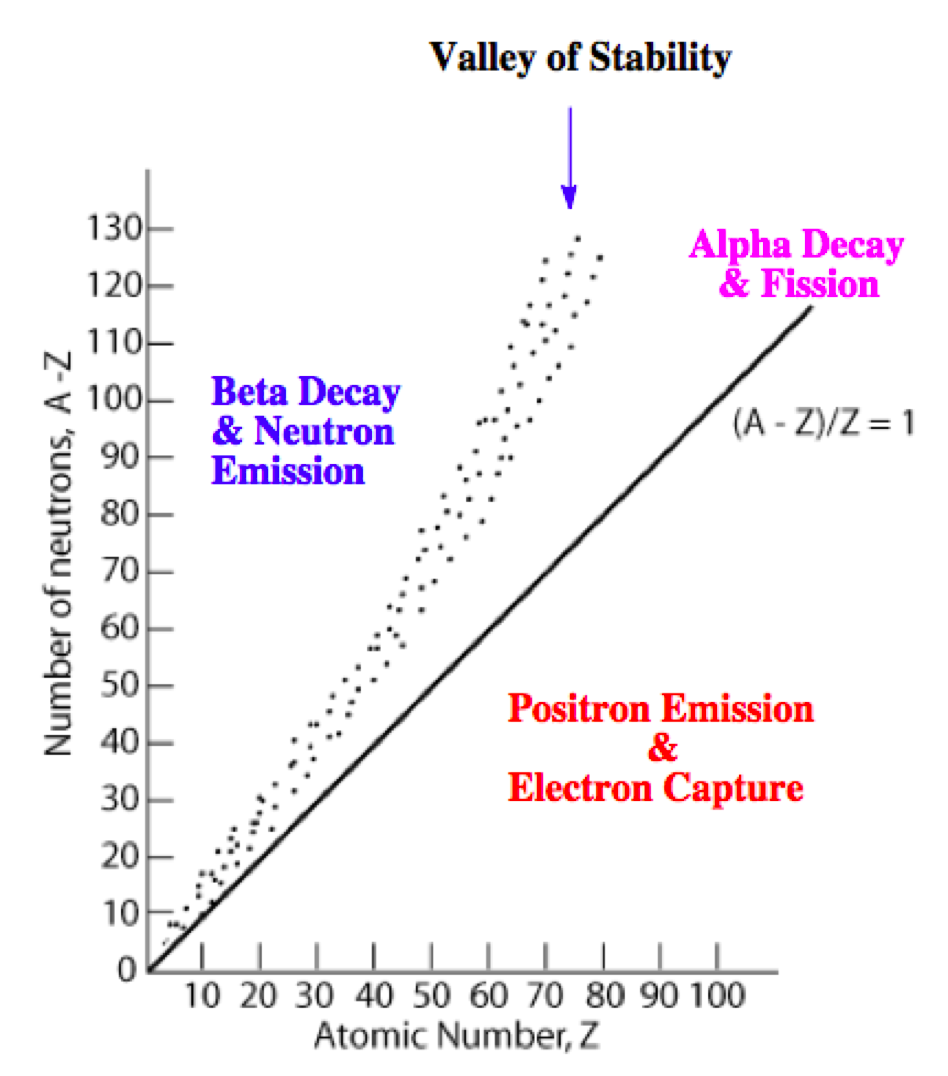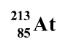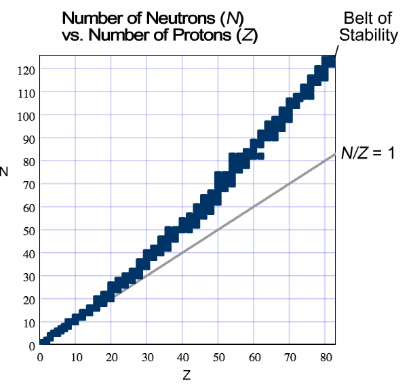# Problem: Which type of nuclear decay is the following radioactive isotope likely to undergo? a) beta decayb) alpha decayc) positron emission or electron captureHintAll known stable isotopes are shown on the graph. The points form what is know as the "belt of stability".If a radioactive isotope is above the belt of stability, think about what type of decay would decrease its N/Z ratio. If an unstable isotope is below the belt of stability, think about what type of decay would increase its N/Z ratio. If a radioactive isotope is off the chart (Z &gt; 83), think about what type of decay would decrease Z the most.

###### FREE Expert Solution

We’re being asked to predict the most likely mode of decay for each of the radioactive isotope given

Recall that the stability of an isotope depends on the ratio of neutrons to protons (N/Z). This means for stable isotopes with:

• Z ≤ 20; the N/Z ratio should be equal to 1.0

• 20 < Z ≤ 40; the N/Z ratio should be equal to 1.25

• 40 < Z ≤ 80; the N/Z ratio should be equal to 1.50

• Z < 83; the stable nuclide does not exist

The N/Z values for stable isotopes form the valley or band of stability:This means for isotopes:

• above the valley of stability: there too many neutrons in the isotope

Mode of decay: Either beta decay (0–1β) or neutron emission (10n; not in the choices)

• below the valley of stability: there too many protons in the isotope

Mode of decay: Either positron emission (01e) or electron capture (0–1e)

• with atomic mass ≥ 200 amu: there too many protons and neutrons in the isotope

Mode of decay: alpha decay (42α)

Recall that the atomic mass is the sum of protons and neutrons and the atomic number tells us the number of protons.

87% (107 ratings)###### Problem Details

Which type of nuclear decay is the following radioactive isotope likely to undergo?a) beta decay

b) alpha decay

c) positron emission or electron captureHint

All known stable isotopes are shown on the graph. The points form what is know as the "belt of stability".

If a radioactive isotope is above the belt of stability, think about what type of decay would decrease its N/Z ratio. If an unstable isotope is below the belt of stability, think about what type of decay would increase its N/Z ratio. If a radioactive isotope is off the chart (Z > 83), think about what type of decay would decrease Z the most.